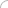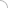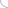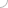SAP transactions

SAP transactions

<<PreviousNext>>

Explain transact
Explain dialog program. What requirements it must fulfill?
List the basic components of a dialog program.
Explain: a.) PBO events b.) PAI events
Explain Dynamic Program and its components.
Explain an ABAP/4 module pool.
Situation - You want to transfer data from field data to screen fields. How would you do that?
Explain GUI status. What are its elements?
Situation - You want to create/ edit a GUI status. How would you do that?
How does a Dynamic Program interact with ABAP/4 modules?
How is the function code handled in flow logic?
List the statements which control the screen flow.
Explain: a.) FIELD statement b.) CHAIN statement c.) ON INPUT FIELD statement d.) ON REQUEST FIELD statement e.) ON *-INPUT statement f.) Conditional chain statement g.) AT EXIT-COMMAND h.) Leave statement
Name the two "next screen" attributes. Which of them takes the priority?
You want to set the navigation to next screen to static/dynamic. Is it possible? If yes, how would you do it?
Explain the following: a.) SET SCREEN b.) CALL SCREEN c.) CALL Mode d.) Dialog module
Explain a.) Logical Unit of WOrk. b.) Update transaction
Situation - You used SET SCREEN command without using LEAVE SCREEN. What would happpen?
What does SCREEN No. 0 mean?
Explain Supress Dialog. What does it do?
Explain the memory table SCREEN. What is its significance? What are the different fields in it?
What attributes of a field can be activated or deactivated at runtime?
Explain Screen groups and its uses.
Explain SUbscreen. What are its uses and restrictions on it?
How would you display a table in a screen?
Explain: a.) TABLE CONTROLS b.) STEP LOOPS
What are the keywords involved in a Dynamic Program?
Explain: a.) PBO events b.) PAI events
You need to code a LOOP statement in both the PBO and PAI events for each table in the screen. Why?
Explain SY-STEPL field.
Situation - You want to declare a table control in the ABAP/4 program. How?
Explain: a.) Step Loops b.) Static Step Loops c.) Dynamic Step Loops
How do you produce a list within a transaction?
When does the current screen processing terminate?
Explain Supress-Dialog command. What is its use?
What would happen if you used Leave to list-processing with Suppress-Dialog?
Situation - A user programmed some transactions. What would you do to protect them?
Explain: a.) Synchronous mode b.) Asynchronous mode
List the modes in which update tasks work.
Explain following integrities in Database: a.) Semantic Integrity b.) Relational Integrity c.) Primary Key Integrity d.) Value Set Integrity e.) Foreign Key Integrity f.) Operational Integrity
Explain SAP Locking. What does a lock object involve?
Explain the following kinds of lock modes: a.) Shared Lock b.) Exclusive Lock c.) Extended exclusive list
Situation - You want to include a lock object in the transaction. How would you do that?
Explain Matchcode.
Situation - You want to get the context sensitive F1 help on a field. How would you do that?
Explain - Roll Area.
What does the system do to handle roll areas for external program components?
What are function modules? Explain the following parameters in the function mocule. a.) EXPORTING b.) IMPORTING c.) TABLES d.) CHANGING
Explain the following types of transactions: a.) Leave transaction b.) Call transaction
What are the various options to pass selection and parameter data to a report?
Situation - You want to print a report rather than seeing it on the screen. How would you do that?
Situation - You want to send some data to external programs. How would you do that?
Explain following parameters of SAP memory: a.) SPA b.) GPA

<<Previous   Next>>Interview questions